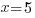### How does it work?

Option 1: Just fill in the form with your homework question, and someone will respond shortly with an answer.

Option 2: Take a photo (or link) of your question, and email or text it to us.

### Like us?# Introduction to Algebra

Algebra is the basis for much of the math taught in high school. Too often, grade 11 or 12 students struggle with simple algebra that they should have mastered in grade 8. Improving your algebra skills is extremely important if you want to succeed in high school and university.

As a general rule, any operation you perform on one side of the equation must also be performed on the other side of the equation. Let’s take a look at some examples, solving for x.We want to isolate for x, or ‘get x by itself’ on the left-hand side (LHS).Subtract 5 from both sides.Note that 5-5=0This was a particularly easy question, and we probably could have answered it by simply asking ourselves, “What number, when added to 5, equals 8?” Of course, 5+3=8, so the number is 3.

Let’s look at a slightly harder example:We want to isolate for x or ‘get it by itself’ on the right-hand side (RHS).Add x to both sides.Subtract 7 from both sides.Do the subtraction 12-7 on the LHS.Multiplication and DivisionThis means 3 multiplied by a number, x, is equal to 21.To isolate for x, we need to divide both sides by 3.Note that 3/3=1, and 1x=x.This means a number, x, divided by 4 is equal to 6.To isolate for x, we need to multiply both sides by 4.Note that 4/4=1.